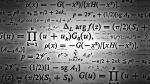# Science Quiz / Almost Useless Science Trivia

Random Science or Biology Quiz

## Can you name the trivia questions about math, chemistry, biology and physics?

#### bybeachybum28  Plays Quiz Updated Aug 14, 2013

Score
0/30
Timer
07:00
Chemistry: What is any one of the alternate names for NaCl?
Math: What number is 1012 in the US but 1018 in the UK?
Physics: Who is the only woman with 2 Nobel Prizes, one in chemistry and one in physics?
Math: What do you call a line segment that connects any two points on a circle?
Chemistry: What is the more common term for
-log([H3O+]) ?
Biology: In the biological classification system, what comes between species and family?
Physics: Which British scientist came up with the 3 Laws of Motion?
Chemistry: What is the non-technical name for dihydrogen monoxide?
Math: Name the sequence that begins 1,1,2,3,5,8,13...
Biology: What is the movement of water through a selectively-permeable membrane?
Physics: Which star is the closest to the planet Earth?
Chemistry: What is the name for 0 Kelvin or -273.15°C?
Math: What trigonometric function is the same as sine divided by cosine?
Biology: Geometrically, what is the specific structure of a DNA molecule called?
Physics: On the electromagnetic spectrum, what type of radiation has the shortest wavelength?
Chemistry: Name any one of the noble gases that isn't radioactive.
Math: What is the more common name for a regular hexahedron?
Earth Science: What is the most abundant element in the Earth's atmosphere?
Physics: What is the SI unit for energy, named after an English physicist?
Chemistry: Complete the other half of the Ideal Gas Law: PV =___
Math: For a pair of points, what does (y2-y1)/(x2-x1), aka rise over run, represent?
Biology: Name either of two bases that completes this set: guanine, cytosine, adenine, ___.
Physics: In E=mc2, what is the name of the constant represented by c?
Biology: What German-speaking friar is famous for discovering the field of genetics?
Chemistry: What process is the opposite of oxidation, where electrons are gained by a species?
Biology: What does a plant cell have surrounding it that an animal cell does not?
Physics: What is the world's largest and highest-energy particle accelerator?
Chemistry: Using element symbols, decode this message: sulfur polonium carbon potassium.
Math: What kind of number has factors that sum to itself like 6 (=1+2+3) or 28(=1+2+4+7+14)?
Biology: What are the hairlike sensory|motion structures on the outside of cells called?

### You're not logged in!

Compare scores with friends on all Sporcle quizzes.
OR